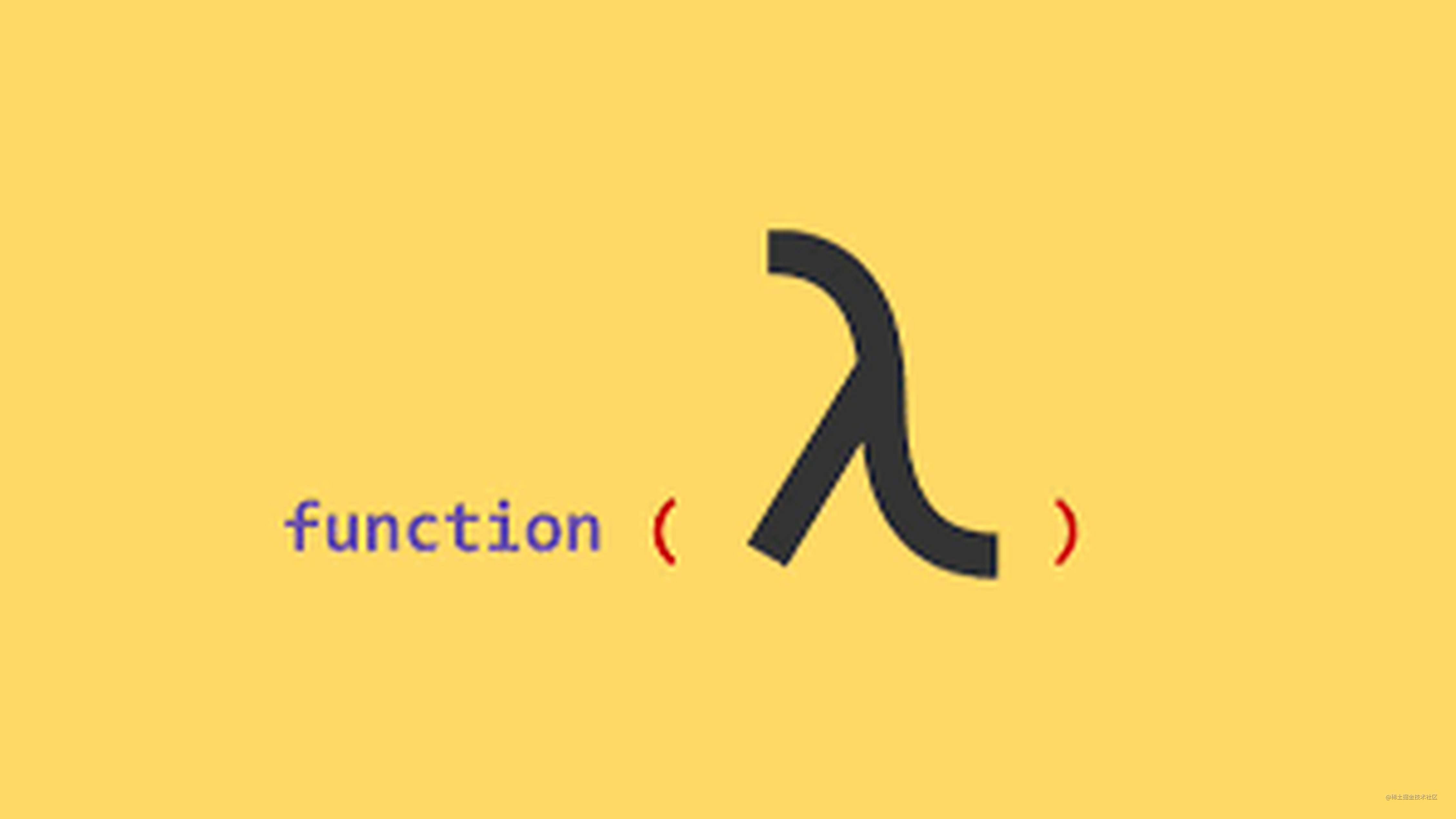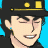# XDM，JS如何函数式编程？看这就够了！（三）## 组合函数

### 含义

``````function words(str) {
return String( str )
.toLowerCase()
.split( /\s|\b/ )
.filter( function alpha(v){
return /^[\w]+\$/.test( v );
} );
}

function unique(list) {
var uniqList = [];

for (let i = 0; i < list.length; i++) {
if (uniqList.indexOf( list[i] ) === -1 ) {
uniqList.push( list[i] );
}
}

return uniqList;
}

var text = "To compose two functions together";

var wordsFound = words( text );
var wordsUsed = unique( wordsFound );

wordsUsed;

//  ["to", "compose", "two", "functions", "together"]
````````````var wordsUsed = unique( words( text ) );

wordsUsed
``````

``````function uniqueWords(str) {
return unique( words( str ) );
}

uniqueWords(text)
````````````uniqueWords <-- unique <-- words <-- text
``````

### 封装盒子

``````function uniqueWords(str) {
return unique( words( str ) );
}

function uniqueWords_A(str) {
return unique_A( words_A( str ) );
}

function uniqueWords_B(str) {
return unique_B( words_B( str ) );
}

...
``````

``````function compose2(fn2,fn1) {
return function composed(origValue){
return fn2( fn1( origValue ) );
};
}

// ES6 箭头函数形式写法
var compose2 =
(fn2,fn1) =>
origValue =>
fn2( fn1( origValue ) );
````````````var uniqueWords = compose2( unique, words );

var uniqueWords_A = compose2( unique_A, words_A );

var uniqueWords_B = compose2( unique_B, words_B );
``````

### 任意组合

``````finalValue <-- func1 <-- func2 <-- ... <-- funcN <-- origValue
````````````function compose(...fns) {
return function composed(result){
// 拷贝一份保存函数的数组
var list = fns.slice();

while (list.length > 0) {
// 将最后一个函数从列表尾部拿出
// 并执行它
result = list.pop()( result );
}

return result;
};
}

// ES6 箭头函数形式写法
var compose =
(...fns) =>
result => {
var list = fns.slice();

while (list.length > 0) {
// 将最后一个函数从列表尾部拿出
// 并执行它
result = list.pop()( result );
}

return result;
};
``````

``````function skipShortWords(list) {
var filteredList = [];

for (let i = 0; i < list.length; i++) {
if (list[i].length > 4) {
filteredList.push( list[i] );
}
}

return filteredList;
}

var text = "To compose two functions together";

var biggerWords = compose( skipShortWords, unique, words );

var wordsUsed = biggerWords( text );

wordsUsed;
// ["compose", "functions", "together"]
``````

``````function skipLongWords(list) { /* .. */ }

var filterWords = partialRight( compose, unique, words ); // 固定 unique 函数 和 words 函数

var biggerWords = filterWords( skipShortWords );
var shorterWords = filterWords( skipLongWords );

biggerWords( text );
shorterWords( text );
``````

filterWords 函数是一个更具有特定功能的变体（根据第一个函数的功能来过滤字符串）。

### compose 变体

compose(..)函数非常重要，但我们可能不会在生产中使用自己写的 compose(..)，而更倾向于使用某个库所提供的方案。了解其底层工作的原理，对我们强化理解函数式编程也非常有用。

``````function compose(...fns) {
// 拿出最后两个参数
var [ fn1, fn2, ...rest ] = fns.reverse();

var composedFn = function composed(...args){
return fn2( fn1( ...args ) );
};

if (rest.length == 0) return composedFn;

return compose( ...rest.reverse(), composedFn );
}

// ES6 箭头函数形式写法
var compose =
(...fns) => {
// 拿出最后两个参数
var [ fn1, fn2, ...rest ] = fns.reverse();

var composedFn =
(...args) =>
fn2( fn1( ...args ) );

if (rest.length == 0) return composedFn;

return compose( ...rest.reverse(), composedFn );
};
``````

``````var biggerWords = compose( skipShortWords, unique, words );

// 变成

var biggerWords = pipe( words, unique, skipShortWords );
``````

``````...
while (list.length > 0) {
// 从列表中取第一个函数并执行
result = list.shift()( result );
}
...
``````

### 抽象能力

``````function saveComment(txt) {
if (txt != "") {
}
}

function trackEvent(evt) {
if (evt.name !== undefined) {
events[evt.name] = evt;
}
}
``````

``````function storeData(store,location,value) {
store[location] = value;
}

function saveComment(txt) {
if (txt != "") {
}
}

function trackEvent(evt) {
if (evt.name !== undefined) {
storeData( events, evt.name, evt );
}
}
``````

``````function conditionallyStoreData(store,location,value,checkFn) {
if (checkFn( value, store, location )) {
store[location] = value;
}
}

function notEmpty(val) { return val != ""; }

function isUndefined(val) { return val === undefined; }

function isPropUndefined(val,obj,prop) {
return isUndefined( obj[prop] );
}

function saveComment(txt) {
}

function trackEvent(evt) {
conditionallyStoreData( events, evt.name, evt, isPropUndefined );
}
``````

``````function getData() {
return [1,2,3,4,5];
}

// 命令式
var tmp = getData();
var a = tmp;
var b = tmp;

// 声明式
var [ a ,,, b ] = getData();
``````

## 阶段小结